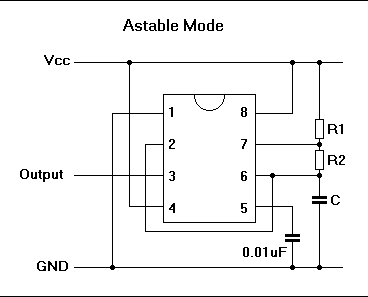# 555定时电路在线计算器

### App说明

TH (高电平时间s) = 0.693 * (R1 + R2) * C

TL (低电平时间s) = 0.693 * R2 * C

Frequency(输频出率) = 1.44 / ((R1 + R2 + R2) * C

DCP(占空比%) = (T(h) / (T(h) + T(l))) * 100### 使用示例

TH (高电平时间s) = 0.693 * (R1 + R2) * C=4.851

TL (低电平时间s) = 0.693 * R2 * C=3.465

Frequency(输频出率) = 1.44 / ((R1 + R2 + R2) * C=0.120

DCP(占空比%) = (T(h) / (T(h) + T(l))) * 100=58.333